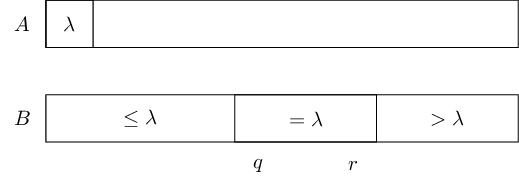# 3-Way Partition solved by 821

Feb. 21, 2014, 4:25 p.m. by Rosalind Team

Topics: Sorting

## Problem

This problem is very similar to “2-Way Partition”, but now the goal is to partition an input array more carefully.Given: A positive integer $n \le 10^5$ and an array $A[1..n]$ of integers from $-10^5$ to $10^5$.

Return: An array $B[1..n]$ such that it is a permutation of $A$ and there are indices $1 \le q \le r \le n$ such that $B[i] < A$ for all $1 \le i \le q-1$, $B[i]=A$ for all $q \le i \le r$, and $B[i] > A$ for all $r+1 \le i \le n$.

## Sample Dataset

9
4 5 6 4 1 2 5 7 4


## Sample Output

2 1 4 4 4 5 7 6 5